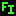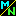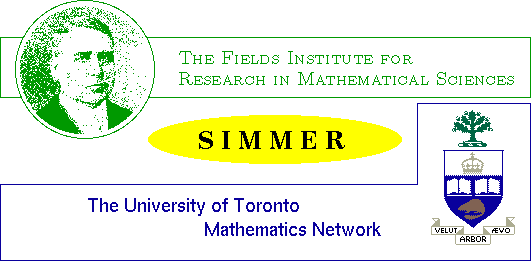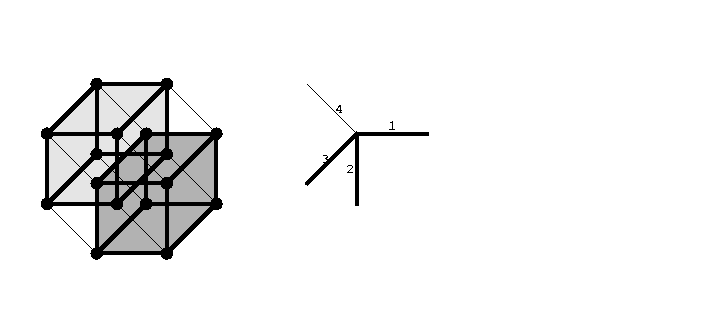Navigation Panel:(These buttons explained below)Society Investigating Mathematical Mind-Expanding Recreations

# January 1998 Feature PresentationHow to See in More DimensionsRagnar-Olaf Buchweitz

Department of Mathematics
University of Toronto

This is a summary of what was presented and discussed at the January 29th SIMMER meeting, along with some problems and questions to think about.

## Introduction

Let us imagine a being restricted to two dimensions, for example an ant-like creature that can crawl in a plane but can never venture into the third dimension, and thus can never move above or below the plane of its existence. How would this being be able to appreciate our universe? A beautiful tale by E. A. Abbott (Flatland: A Romance of many Dimensions, by A Square, Boston, 1885; 6th edition reprinted by Dover Publications 1952), a schoolmaster of the classics--not mathematics!--explored this theme more than a hundred years ago, at a time when Einstein was four years old and space-time or the fourth dimension were at best occult notions.

As W. W. Rouse Ball put it in Mathematical Recreations and Essays, 9th edition, 1920, p.426 (according to H. S. M. Coxeter in Geometry, Toronto 1961, p. 397):

If an inhabitant of flatland was able to move in three dimensions, he would be credited with supernatural powers by those who were unable so to move; for he could appear or disappear at will, could (so far as they could tell) create matter or destroy it. . . We may go one step lower, and conceive of a world of one dimension--like a long tube--in which the inhabitants could move only forwards and backwards. . . Life in line-land would seem somewhat dull. . . An inhabitant could only know two other individuals; namely, his neighbours, one on each side.
Here we wish to go into the other direction: What will life be like in a four-dimensional world where we can at ease leave our three dimensions, go through the fourth and then reappear at another place? To quote Coxeter directly (from Regular Polytopes, 3rd edition, Dover Publications 1973):
Only one or two people have ever attained the ability to visualize hyper-solids as simply and naturally as we ordinary mortals visualize solids; but a certain facility in that direction may be acquired by contemplating the analogy between one and two dimensions, then two and three, and so (by a kind of extrapolation) three and four. (p. 119)
and in the introduction he writes:
As for . . . figures in four or more dimensions, we can never fully comprehend them by direct observation. In attempting to do so, however, we seem to peep through a chink in the wall of our physical limitations, into a world of dazzling beauty.
(And Coxeter himself widened that chink considerably! He came to the University of Toronto more than 60 years ago; his 90th birthday last year is responsible for the sculpture of Borromean rings that you can see at the entrance to the Fields Institute.)

But it is not only aesthetic pleasure, there are practical applications of four (or higher) dimensional thinking as well. Be it hyper-cubes modelling optimal networks for the flow of information in parallel processing computers; or zonohedra, projections of higher dimensional objects, that play an important role in design and architecture as "they can be expanded, contracted, or distorted by altering" the projection (see W. D. Hillis: "The Connection Machine", Scientific American, June 1987; J. Kappraff, Connections, The Geometric Bridge between Arts and Science, McGraw-Hill Inc. 1991).

Aside from looking at projections, we treat a simple practical application, taken from Kappraff's book: Sir William Hamilton, the discoverer of quaternions and godfather of Hamiltonian systems in Physics, asked in 1862 to find on a given road map, or graph, a route which will pass once and only once through every city on the map. He phrased it more formally though and added further constraints to make it tougher. It turns out that such a route or Hamiltonian path that visits exactly once every corner of the hypercube is intimately related to a winning strategy in the game of Towers of Hanoi.

By the way, Sir Hamilton wanted, and found, a route that passes through all corners, or vertices, of a regular dodecahedron. He presented it in a lecture to the British Association in Dublin in 1857. Can you find such a route as well? Can you find the simplest road map on which it is not possible to visit every city exactly once? Hamiltonian paths or circuits are of great interest in many applications, but until this day no efficient rule or recipe is known that decides whether a given map or graph allows such circuits--of course you may always "just" try all possible routes.

But back to the fourth dimension.

## An Experiment

The first problem is meant to make you aware of properties of shadows of an ordinary cube. This exercise should help to understand what happens later on!

Problem 1: Build several cubes from cardboard. Push a pencil or a stiff wire through opposite vertices(corners) of a cube and place the cube in front of a vertical white wall or a white sheet of paper. Now shine the beam of a flashlight horizontally onto the cube (the closer the cube to the white surface and the further the flashlight from the cube the better, but that might depend upon the length of your arms!) The following picture should explain it:(a)
Rotate the cube slowly and record the shapes of the shadow the cube casts.

What kinds of polygons do you get?

(b)
How do your observations change if you hold the pencil horizontally, in the direction of the light, and rotate then?

The shadow you observe is the "vertex first projection" of the cube.

(This is more challenging as your fingers tend to obscure things. Can you think of a way to keep your fingers out of the picture?)

(c)
Take the same or a second cube and this time push the pencil or wire through the midpoints of two opposite edges.

Record again what you observe.

(d)
Repeat the experiment with the pencil pushed through centres of opposite faces.

## Constructing a 4-Cube or Tesseract

Now we come to our main topic. We want to study projections of four- or higher-dimensional objects, in particular of the four-dimensional cube or Tesseract. To get an idea what we are talking about, let us first construct the Tesseract one dimension at a time:

We begin with a point that we might call a 0-cube as no dimensions are yet involved.

Then we choose our first dimension, say horizontal, and displace the 0-cube or point one unit in that direction, connecting the starting point and the endpoint. What we get is a line segment or 1-cube.

Now we find the first interesting shape: Choose a direction or dimension perpendicular to the first one and displace the 1-cube one unit in the new direction ("down" in our picture), recording the path of each vertex. What we end up with, is clearly a square or 2-cube. To keep track of our dimensions, we display as well the vector star displaying the directions or dimensions chosen.If we want to continue, we encounter the first difficulty: we need to choose a third direction, perpendicular to the first two, whence we have to move out of the paper plane or computer screen. But our brain is so used to the three-dimensional world that it constructs the correct image from the plane projection! Moving the square in a southwest direction creates the image of the square moving towards us from the screen. Recording again the paths of each vertex, we obtain all the edges of an ordinary 3-cube:Note that the visual clues are not quite sufficient for our eyes or brain to decide whether the grey square should be in front or in the back and accordingly the cube appears in two different ways: looking at the picture for some time, the grey square jumps in and out. Now we confuse our brain even more, by moving the cube into the fourth dimension! We choose a fourth direction, that should be perpendicular to the three given ones, whence we now leave the physical world. It should help to visualize the following two-dimensional projection of the 4-cube or Tesseract as a movie: the dark grey cube moves in time along the fourth direction, chosen here to point northwest. Its future end position is shown in light grey.In the following picture we have repeated Problem 1 for the Tesseract, and we have encoded the different dimensions by four colours: A "four-dimensional flashlight" has been used to project it parallel to a diagonal joining two opposite vertices into 3-space, a "vertex first projection".Problem 2: What kind of polyhedron forms the outer shell of the projection? (How many vertices, what kind of faces and how many of them? How many faces meet in a vertex?)

The vertices sit on 3 concentric spheres if one counts the centre as a sphere.

Which vertices sit on the outermost sphere and what kind of polyhedron do you get when connecting them by wires?

Same question for the vertices on the second sphere.

## Take a Walk along the Edges

To explore the geometric objects we introduced so far, let's take a walk. The aim is to visit all vertices exactly once by walking along edges (not all edges will be needed!) Such a walk describes a Hamiltonian Path in the graph defined by the vertices and edges.

For the 1-cube or line segment, once we choose the starting point, there are no further choices: just walk along the only edge to the other vertex.

If we start at the top left corner of a square, we can walk first along the first dimension, that is horizontally, then down along the second dimension, and finally back along the first dimension again.

If we follow the same recipe for the cube, we have by now visited all vertices of the back face, say, and are standing at the lower left corner. If we move along the third dimension we get to the front face, and here we simply reverse the path we took on the back face.

The same procedure works for the 4-cube and the pictures below show the described Hamiltonian walk alongside the 2,3,4-cube respectively. As numbering the edges becomes too confusing, we colour-code again the edges of the Tesseract: Yellow for the first dimension, green for the second, blue for the third and red for the fourth.Problem 3: (As learned from J. Kappraff, Connections. The Geometric Bridge between Art and Science, McGraw-Hill, Inc., 1991)

Play the game "Tower of Hanoi" with 1, 2, 3 and finally 4 discs.

What is the relationship between solutions to that game and the indicated Hamiltonian paths in an n-cube?

Problem 3': When following the Hamiltonian path, how often do you walk along an edge in the first dimension (horizontal, yellow), how often along one in the second dimension (vertical, green), and so on? How many edges do you have to have in total in a Hamiltonian path on an n-cube?

Substituting the actual numbers into the formula

#(edges of first dimension) + #(edges of second dimension) + . . . = #(all edges in path)
what kind of identity do you get?

(But it is not due to this example that one talks of "geometric series".)

## The Fifth Dimension

We have difficulties visualizing even four dimensions, but one of the beauties of mathematics is its generalization from the known! If you want to think in terms of physical dimensions, you might envision length, width, height, time and, say, temperature as your five directions/dimensions.

Problem 4: The picture below shows a highly symmetric (though slightly distorted) plane projection of a 5-cube and its vector star.

(a)
Draw onto (a copy of) it the Hamiltonian path corresponding to the solution of the "Tower of Hanoi" game with 5 disks.

(b)
A square is bounded by four sides, a cube by six squares. How many cubes form the boundary of a tesseract? How many tesseracts form the boundary of a 5-cube?

(c)
Shade one of the bounding tesseracts in the projection of the 5-cube and show that by sliding it in a fifth direction you can obtain the full 5-cube. (Remember or revisit the step-by-step construction of the Tesseract.)

How can you find all of the Tesseracts in the picture?Problem 5: In the given plane projection of the 5-cube, the vertices are distributed on four concentric circles if one counts the centre as a circle.

Count all vertices. Do you get the correct number? What has happened?

How much of the edges has been lost in this projection?

Can you draw a slightly distorted version that shows all the edges and vertices clearly without overlaps (but intersections are of course unavoidable)?

Problem 6: The perimeter of the above plane projection is a regular decagon, the perimeter of the plane projection of the tesseract we saw earlier is a regular octagon (go and look again).

If you did your experiments in Problem 1, you should have no difficulties to draw a projection of a cube whose perimeter is a regular ?-gon where the number ? of vertices is as large as possible. Draw also a slightly distorted version in which there are no overlaps of vertices or edges.

Problem 7: We just studied (very) regular polygons that appear as perimeters of plane projections of n-cubes. The edges projecting onto such a perimeter correspond to a closed(!) walk on the n-cube, called a Petrie polygon. Paying attention to the sequence of colours/dimensions, can you formulate a rule for describing such Petrie polygons? These walks are the closest thing to a great circle on an n-cube. How many different such great circles or Petrie polygons are there on an ordinary cube, a tesseract, an n-cube? How many of them pass through a fixed vertex? Can you draw a second such walk in the regular projections of the 3,4,5-cube respectively?

Navigation Panel: